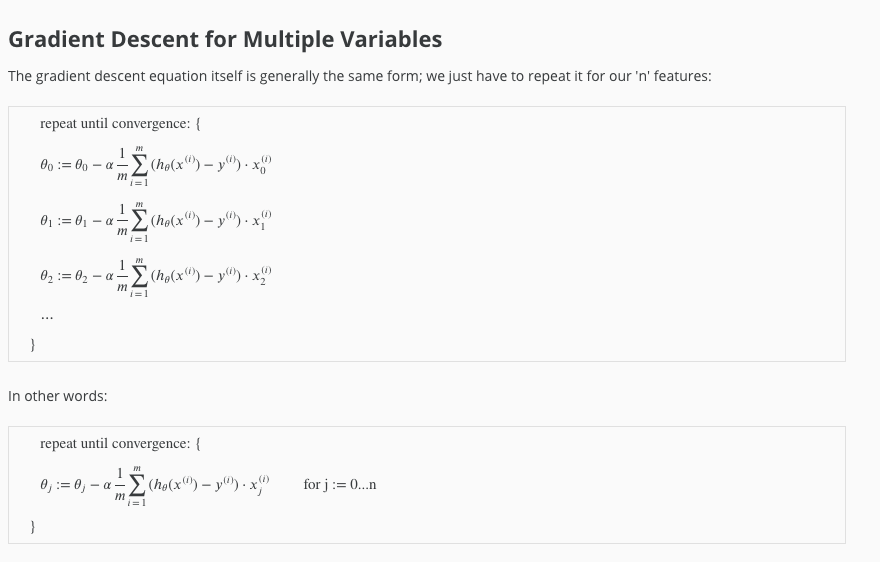##### hypothesis function
• Multiple features, single out come variables.
• Definition of hypothesis function
• hθ(x) = θ0 + θ1x1 + θ2x2 … + θnxn
• x0 = 1
• hθ(x) = θ0x0 + θ1x1 + θ2x2 … + θnxn
• x = (N+1) vector, θ = (N+1) vetor
• hθ(x) = θTx
##### Multivariate linear regression##### Feature scaling
• If ranges for various features vary quite a bit, leads to skewed chart, leading to slow rate of convergence.
• To speed up speed of convergence scale all features so that ranges for all are between 0 to 1.
• Example - Feature 1 = potential values 0 to 10000. ScaledFeature 1 = (Feature 1)/10000 = potential values 0 to 1.
• If features can have negative values then potential values will be -1 to 1
##### Mean normalization
• All features having similar mean (zero) helps with rate of convergence.
• Normalize all features so that the mean is at zero.
##### Formula to do feature scaling and mean normalization

xi = (xii)/Si

• &mui = average value of the feature.
• Si = Max Value - Min Value for the feature
• Features a range of -0.5 to 0.5
##### Practical tips for gradient descent
• Best way to debug if gradient descent is working is by plotting J(θ) for number of iterations.
• Learning rate too small = slow convergence
• Learning rate too high = J(θ) may not converge.
• Try α = 0.001, 0.003, 0.01, 0.03, 0.1, 0.3, 1…
##### Polynomial regressionθ = (XTX)-1XTy